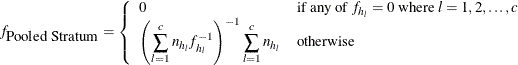The SURVEYREG Procedure

Computational Details

Subsections:

Notation

For a stratified clustered sample design, observations are represented by an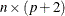matrix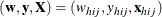where

•denotes the sampling weight vector

•denotes the dependent variable

•denotes the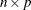design matrix. (When an effect contains only classification variables, the columns ofthat correspond this effect contain only 0s and 1s; no reparameterization is made.)

•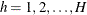is the stratum index

•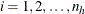is the cluster index within stratum h

•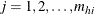is the unit index within cluster i of stratum h

• p is the total number of parameters (including an intercept if the INTERCEPT effect is included in the MODEL statement)

•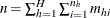is the total number of observations in the sample

Also,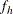denotes the sampling rate for stratum h. You can use the TOTAL= or RATE= option to input population totals or sampling rates. See the section Specification of Population Totals and Sampling Rates for details. If you input stratum totals, PROC SURVEYREG computesas the ratio of the stratum sample size to the stratum total. If you input stratum sampling rates, PROC SURVEYREG uses these values directly for. If you do not specify the TOTAL= or RATE= option, then the procedure assumes that the stratum sampling ratesare negligible, and a finite population correction is not used when computing variances.

Regression Coefficients

PROC SURVEYREG solves the normal equations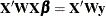by using a modified sweep routine that produces a generalized (g2) inverse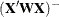and a solution (Pringle and Rayner 1971)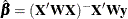where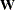is the diagonal matrix constructed from WEIGHT variable values.

For models with CLASS variables, there are more design matrix columns than there are degrees of freedom (df) for the effect. Thus, there are linear dependencies among the columns. In this case, the parameters are not estimable; there is an infinite number of least squares solutions. PROC SURVEYREG uses a generalized (g2) inverse to obtain values for the estimates. The solution values are not displayed unless you specify the SOLUTION option in the MODEL statement. The solution has the characteristic that estimates are zero whenever the design column for that parameter is a linear combination of previous columns. (In strict terms, the solution values should not be called estimates.) With this full parameterization, hypothesis tests are constructed to test linear functions of the parameters that are estimable.

Design Effect

If you specify the DEFF option in the MODEL statement, PROC SURVEYREG calculates the design effects for the regression coefficients. The design effect of an estimate is the ratio of the actual variance to the variance computed under the assumption of simple random sampling: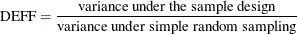See Kish (1965, p. 258) for more details. PROC SURVEYREG computes the numerator as described in the section Variance Estimation. And the denominator is computed under the assumption that the sample design is simple random sampling, with no stratification and no clustering.

To compute the variance under the assumption of simple random sampling, PROC SURVEYREG calculates the sampling rate as follows. If you specify both sampling weights and sampling rates (or population totals) for the analysis, then the sampling rate under simple random sampling is calculated as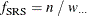where n is the sample size and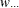(the sum of the weights over all observations) estimates the population size. If the sum of the weights is less than the sample size,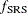is set to zero. If you specify sampling rates for the analysis but not sampling weights, then PROC SURVEYREG computes the sampling rate under simple random sampling as the average of the stratum sampling rates: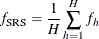If you do not specify sampling rates (or population totals) for the analysis, then the sampling rate under simple random sampling is assumed to be zero: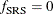Stratum Collapse

If there is only one sampling unit in a stratum, then PROC SURVEYREG cannot estimate the variance for this stratum for the Taylor series method. To estimate stratum variances, by default the procedure collapses, or combines, those strata that contain only one sampling unit. If you specify the NOCOLLAPSE option in the STRATA statement, PROC SURVEYREG does not collapse strata and uses a variance estimate of zero for any stratum that contains only one sampling unit.

Note that stratum collapse only applies to Taylor series variance estimation (the default method, also specified by VARMETHOD=TAYLOR). The procedure does not collapse strata for BRR or jackknife variance estimation, which you request with the VARMETHOD=BRR or VARMETHOD=JACKKNIFE option.

If you do not specify the NOCOLLAPSE option for the Taylor series method, PROC SURVEYREG collapses strata according to the following rules. If there are multiple strata that contain only one sampling unit each, then the procedure collapses, or combines, all these strata into a new pooled stratum. If there is only one stratum with a single sampling unit, then PROC SURVEYREG collapses that stratum with the preceding stratum, where strata are ordered by the STRATA variable values. If the stratum with one sampling unit is the first stratum, then the procedure combines it with the following stratum.

If you specify stratum sampling rates by using the RATE=SAS-data-set option, PROC SURVEYREG computes the sampling rate for the new pooled stratum as the weighted average of the sampling rates for the collapsed strata. See the section Computational Details for details. If the specified sampling rate equals 0 for any of the collapsed strata, then the pooled stratum is assigned a sampling rate of 0. If you specify stratum totals by using the TOTAL=SAS-data-set option, PROC SURVEYREG combines the totals for the collapsed strata to compute the sampling rate for the new pooled stratum.

Sampling Rate of the Pooled Stratum from Collapse

Assuming that PROC SURVEYREG collapses single-unit strata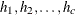into the pooled stratum, the procedure calculates the sampling rate for the pooled stratum as